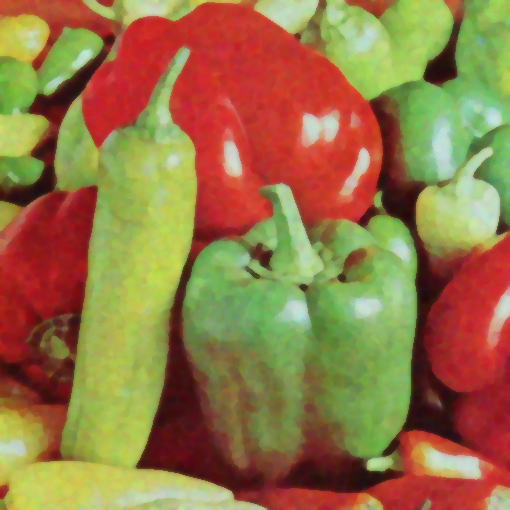## KUWAHARA

 Applies a Kuwahara type edge preserving noise reduction filter to an image.

 USAGE: kuwahara [-d dimension] [-m method] [-s smoothing] infile outfile USAGE: kuwahara [-h or -help] -d ... dimension ... square window dimension; integer>=2; default=3 -m ... method ...... method of processing; 1=mean, 2=gaussian mean, 3=median .................... default=2 -s ... smoothing ... smoothing factor for method 2; float>=1; nominal .................... between 1 and 2; default=1 PURPOSE: To apply a Kuwahara type edge preserving noise reduction filter to an image. DESCRIPTION: KUWAHARA applies a Kuwahara type edge preserving noise reduction filter to an image. The basic Kuwahara filter does the following. For each pixel in the image, the four diagonal neighboring square sized windows (northwest, northeast, southeast and soutwest) that overlap with the pixel at their corners are processed to get their mean and standard deviation. The output image has the corresponding pixel filled with the mean of the four window that has the smallest standard deviation, i.e. the smoothest window. I have modified this approach in several ways. First, I use the eight neighboring windows that overlap the pixel coordinate (northwest, north, northeast, east, southeast, south, southwest and west). Second, I allow three different methods to compute the mean and standard deviation. Method 1 uses a square uniformly weighted mean and corresponding standard deviation. This is the traditional approach. Method 2 uses a circular Gaussian weighted mean within the square window and corresponding standard deviation. Method 3 uses the circular Gaussian weighted approach to compute the standard deviation, but uses a square window median value. Arguments: -d dimension ... DIMENSION is the square window dimension. Values are integer>=2. Nominal values are in the range of 2 to 4. The default=3. Values larger than 4 may cause jagged, flat regions. -m method ... METHOD is the method of processing. The choices are: 1=mean, 2=gaussian mean, 3=median. The default=2. -s smoothing ... SMOOTHING is the smoothing factor for method 2 only. Values are float>=1. Nominal values are between 1 and 2. The default=1. The larger the value the more smoothing. References: http://en.wikipedia.org/wiki/Kuwahara_filter CAVEAT: No guarantee that this script will work on all platforms, nor that trapping of inconsistent parameters is complete and foolproof. Use At Your Own Risk.

### EXAMPLES

 Example 1 -- Variation In Dimension (For Different Methods) Original Image convert lena.png -seed 100 -attenuate 1 +noise gaussian lena_gnoise1s100Arguments: -d 2 -m 1 Arguments: -d 3 -m 1 Arguments: -d 4 -m 1Arguments: -d 2 -m 2 Arguments: -d 3 -m 2 Arguments: -d 4 -m 2Arguments: -d 2 -m 3 Arguments: -d 3 -m 3 Arguments: -d 4 -m 3Example 2 -- Variation Smoothing Original ImageArguments: -d 3 -m 2 -s 1 Arguments: -d 3 -m 2 -s 1.5 Arguments: -d 3 -m 2 -s 2Example 2 -- Variation In Dimension ( actual images sizes are 512 -- click on image to open at full size) Original Image Gaussian Noise Added Image convert peppers.png -seed 100 -attenuation 2 +noise gaussian peppers_colornoise_gauss2.pngArguments: -d 2 -m 2 Arguments: -d 3 -m 2 Arguments: -d 4 -m 2Arguments: -d 5 -m 2 Arguments: -d 6 -m 2 Arguments: -d 7 -m 2What the script does is as follows: Creates a grayscale version of the image Processes the grayscale image for standard deviation at each pixel Processes the color image for mean at each pixel Creates an offset version of the mean and standard deviation images for each appropriate window offset Finds the appropriate offset image that has the lowest standard deviation Gets the mean value corresponding to the same offset and writes that value to the output image at the same location as the input pixel See the script for code details